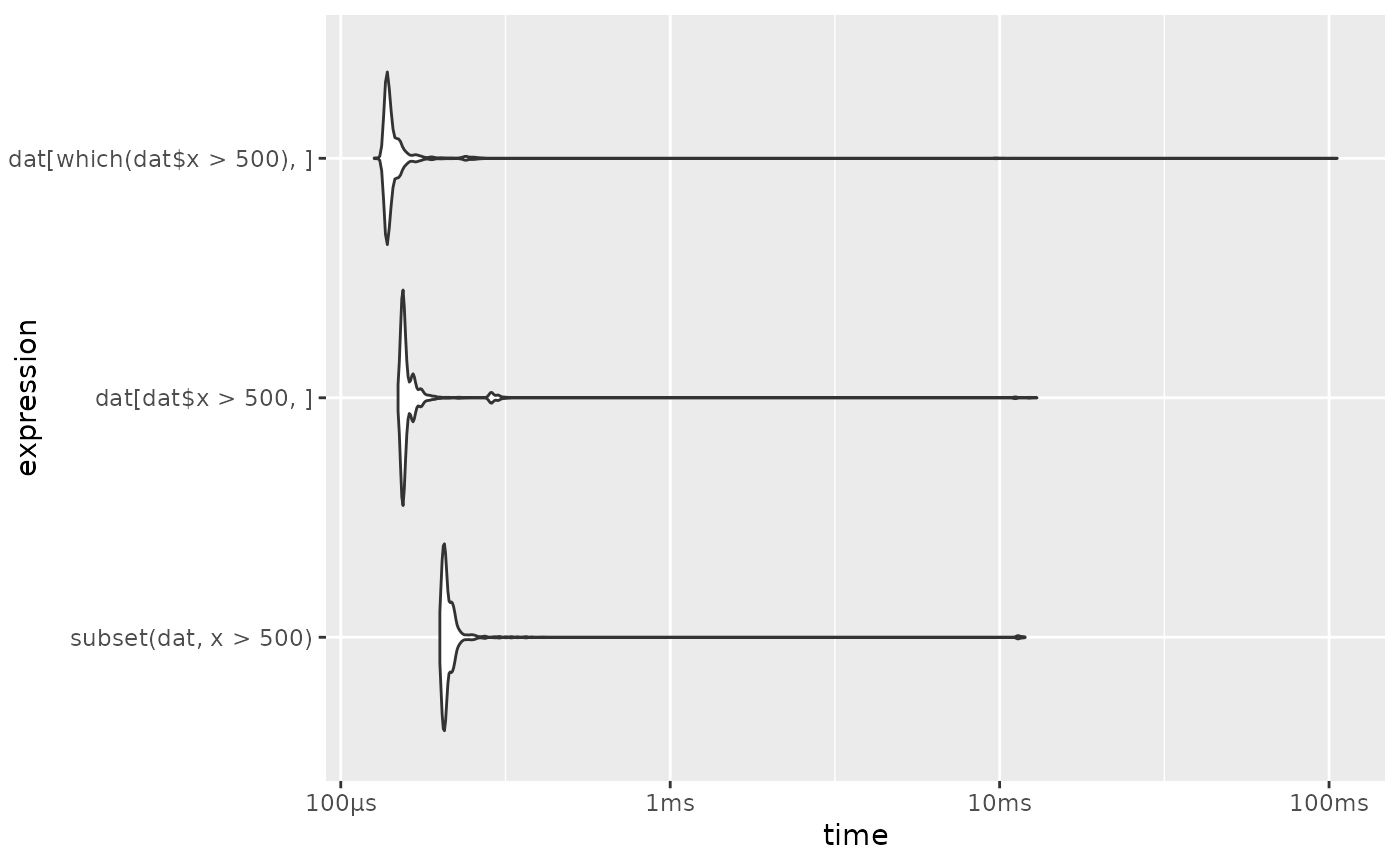Autoplot method for bench_mark objects

autoplot.bench_mark(
object,
type = c("beeswarm", "jitter", "ridge", "boxplot", "violin"),
...
)

# S3 method for bench_mark
plot(x, ..., type = c("beeswarm", "jitter", "ridge", "boxplot", "violin"), y)

## Arguments

object A bench_mark object. The type of plot. Plotting geoms used for each type are beeswarm - ggbeeswarm::geom_quasirandom() jitter - ggplot2::geom_jitter() ridge - ggridges::geom_density_ridges() boxplot - ggplot2::geom_boxplot() violin - ggplot2::geom_violin() Additional arguments passed to the plotting geom. A bench_mark object. Ignored, required for compatibility with the plot() generic.

## Details

This function requires some optional dependencies. ggplot2, tidyr, and depending on the plot type ggbeeswarm, ggridges.

For type of beeswarm and jitter the points are colored by the highest level garbage collection performed during each iteration.

For plots with 2 parameters ggplot2::facet_grid() is used to construct a 2d facet. For other numbers of parameters ggplot2::facet_wrap() is used instead.

## Examples

dat <- data.frame(x = runif(10000, 1, 1000), y=runif(10000, 1, 1000))

res <- bench::mark(
dat[dat$x > 500, ], dat[which(dat$x > 500), ],
subset(dat, x > 500))

if (require(ggplot2) && require(tidyr)) {

# Beeswarm plot
autoplot(res)

# ridge (joyplot)
autoplot(res, "ridge")

# If you want to have the plots ordered by execution time you can do so by
# ordering factor levels in the expressions.
if (require(dplyr) && require(forcats)) {

res %>%
mutate(expression = forcats::fct_reorder(as.character(expression), min, .desc = TRUE)) %>%
as_bench_mark() %>%
autoplot("violin")
}
#>     intersect, setdiff, setequal, union#> Loading required package: forcats